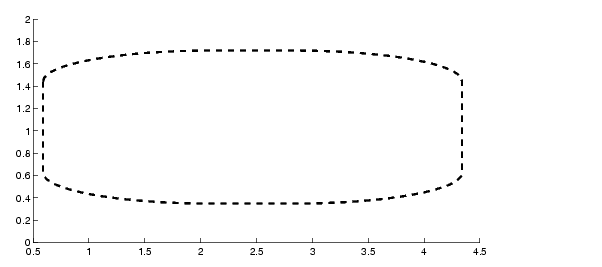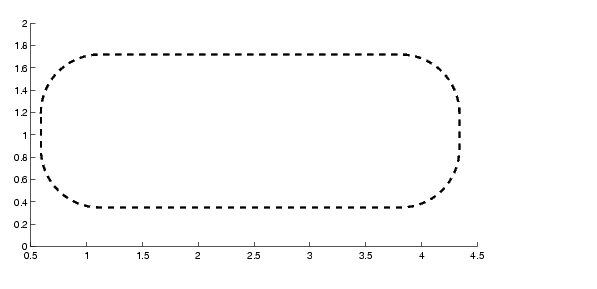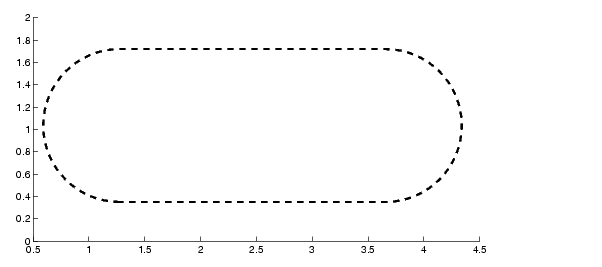MATLAB Function Referencerectangle

Create a 2-D rectangle object

Syntax

• ```rectangle
rectangle('Position',[x,y,w,h])
rectangle(...,'Curvature',[x,y])
h = rectangle(...)
```

Description

`rectangle` draws a rectangle with `Position` `[0,0,1,1]` and `Curvature` `[0,0]` (i.e., no curvature).

`rectangle('Position',[x,y,w,h])` draws the rectangle from the point `x`,`y` and having a width of `w` and a height of `h`. Specify values in axes data units.

Note that, to display a rectangle in the specified proportions, you need to set the axes data aspect ratio so that one unit is of equal length along both the x and y axes. You can do this with the command `axis` `equal` or `daspect``([1,1,1])`.

`rectangle(...,'Curvature',[x,y])` specifies the curvature of the rectangle sides, enabling it to vary from a rectangle to an ellipse. The horizontal curvature `x` is the fraction of width of the rectangle that is curved along the top and bottom edges. The vertical curvature `y` is the fraction of the height of the rectangle that is curved along the left and right edges.

The values of `x` and `y` can range from `0` (no curvature) to `1` (maximum curvature). A value of `[0,0]` creates a rectangle with square sides. A value of `[1,1]` creates an ellipse. If you specify only one value for `Curvature`, then the same length (in axes data units) is curved along both horizontal and vertical sides. The amount of curvature is determined by the shorter dimension.

`h = rectangle(...)` returns the handle of the rectangle object created.

Remarks

Rectangle objects are 2-D and can be drawn in an axes only if the view is `[0 90]` (i.e., `view(2)`). Rectangles are children of axes and are defined in coordinates of the axes data.

Examples

This example sets the data aspect ratio to `[1,1,1]` so that the rectangle displays in the specified proportions (`daspect`). Note that the horizontal and vertical curvature can be different. Also, note the effects of using a single value for `Curvature`.

• ```rectangle('Position',[0.59,0.35,3.75,1.37],...
'Curvature',[0.8,0.4],...
'LineWidth',2,'LineStyle','--')
daspect([1,1,1])```

Specifying a single value of `[0.4]` for `Curvature` produces:

• ``````

A `Curvature` of `` produces a rectangle with the shortest side completely round:

• ``````

This example creates an ellipse and colors the face red.

• ```rectangle('Position',[1,2,5,10],'Curvature',[1,1],...
'FaceColor','r')
daspect([1,1,1])
xlim([0,7])
ylim([1,13])```

`line`, `patch`, rectangle properties

Object Creation Functions for related functions

Object HierarchySetting Default Properties

You can set default rectangle properties on the axes, figure, and root levels.

• ```set(0,'DefaultRectangleProperty',PropertyValue...)
set(gcf,'DefaultRectangleProperty',PropertyValue...)
set(gca,'DefaultRectangleProperty',PropertyValue...)
```

Where `Property` is the name of the rectangle property whose default value you want to set and `PropertyValue` is the value you are specifying. Use `set` and `get` to access the surface properties.

Property List

The following table lists all rectangle properties and provides a brief description of each. The property name links take you to an expanded description of the properties.

 Property Name Property Description Property Value Defining the Rectangle Object `Curvature` Degree of horizontal and vertical curvature Value: two-element vector with values between `0` and `1`Default: `[0,0]` `EraseMode` Method of drawing and erasing the rectangle (useful for animation) Values: `normal`, `none`, `xor`, `background`Default: `normal` `EdgeColor` Color of rectangle edges Value: `ColorSpec` or `none`Default: `ColorSpec [0,0,0]` `FaceColor` Color of rectangle interior Value: `ColorSpec` or `none`Default: `none` `LineStyle` Line style of edges Values: -, --, `:`, -`.`,` none`Default: - `LineWidth` Width of edge lines in points Value: scalarDefault: `0.5` points `Position` Location and width and height of rectangle Value: [`x`,`y`,`width`,`height`]Default: `[0,0,1,1]` General Information About Rectangle Objects `Children` Rectangle objects have no children `Parent` Axes object Value: handle of axes `Selected` Indicate if the rectangle is in a "selected" state. Value: `on`, `off` Default: `off` `Tag` User-specified label Value: any string Default:` '' `(empty string) `Type` The type of graphics object (read only) Value: the string `'rectangle'` `UserData` User-specified data Value: any matrixDefault:` [] `(empty matrix) Properties Related to Callback Routine Execution `BusyAction` Specify how to handle callback routine interruption Value: `cancel`, `queue` Default: `queue` `ButtonDownFcn` Define a callback routine that executes when a mouse button is pressed on over the rectangle Value: string or function handleDefault: `'' `(empty string) `CreateFcn` Define a callback routine that executes when a rectangle is created Value: string or function handleDefault: `'' `(empty string) `DeleteFcn` Define a callback routine that executes when the rectangle is deleted (via `close` or `delete`) Values: string or function handleDefault: `'' `(empty string) `Interruptible` Determine if callback routine can be interrupted Values: `on`, `off` Default: `on` (can be interrupted) `UIContextMenu` Associate a context menu with the rectangle Values: handle of a Uicontextmenu Controlling Access to Objects `HandleVisibility` Determines if and when the rectangle's handle is visible to other functions Values: `on`, `callback`, `off`Default: `on` `HitTest` Determines if the rectangle can become the current object (see the Figure `CurrentObject` property) Values: `on`, `off` Default: `on` Controlling the Appearance `Clipping` Clipping to axes rectangle Values: `on`, `off`Default: `on` `SelectionHighlight` Highlight rectangle when selected (`Selected` property set to `on`) Values: `on`, `off` Default: `on` `Visible` Make the rectangle visible or invisible Values: `on`, `off` Default: `on`record rectangle properties